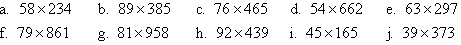Year 7 Interactive Maths - Second Edition

## Projects

### Project 1.1  Napier's Bones (or Rods)

A Scottish mathematician named John Napier (1550-1617 AD) discovered logarithms and invented a simple calculator to multiply any two numbers.  The simple calculator is known as Napier's bones or Napier's rods because it can be constructed from bone, paper etc.

To make Napier's rods (or bones), you need ten strips of paper each divided into 9 parts, one for each of the first 9 multiples of the number at the top of strip as shown below.In each strip, a slanting line separates the tens digit from the units digit.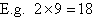This is written as shown in the above diagram.  The slanting line separates the tens digit on the left, 1, from the units digit on the right, 8.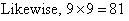The slanting line separates the tens digit on the left, 8, from the units digit on the right, 1.

Copy the strips exactly as shown above, and fill in the blanks.

We can use Napier's bones to multiply any two numbers.  E.g. For 75 × 3, place the multiplier, the '7' and the '5' bone side by side (for 75) as shown below.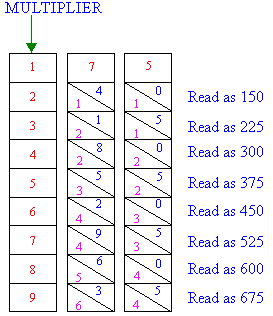To use the above Napier's bones to calculate 3 × 75, sum the numbers along the slanted diagonals of the combined bones using the row applying to the multiplier.  For the two bones, when reading from right to left there are three diagonals which represent units, tens and hundreds when the multiplier is three.  That is the 5 represents 5 units, the 1+1=2 represents 2 tens, and the 2 represents 2 hundreds. Adding 5 units, 2 tens and 2 hundreds gives 225.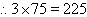Similarly, we find that: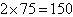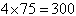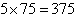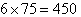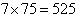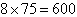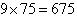1. Use Napier's bones to calculate: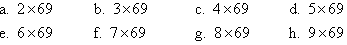2. Use Napier's bones to calculate: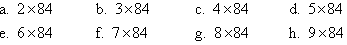3. Use Napier's bones to calculate: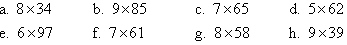To calculate 83 × 24, place the multiplier and the bones for the number being multiplied (i.e. the '8' and '3' bones for 83) side by side as shown below. To find the product first multiply by 4 and then multiply by 20, and add just as you would for long multiplication to find the answer.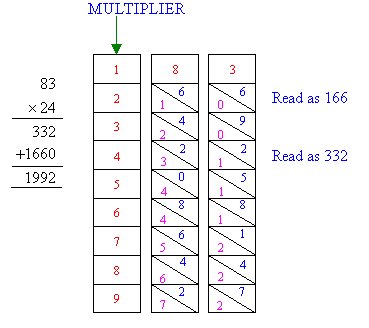4. Use Napier's bones to calculate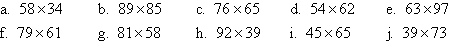5. Use Napier's bones to calculate: